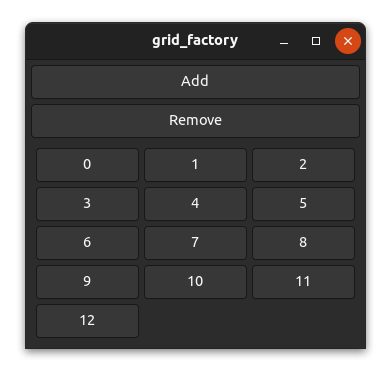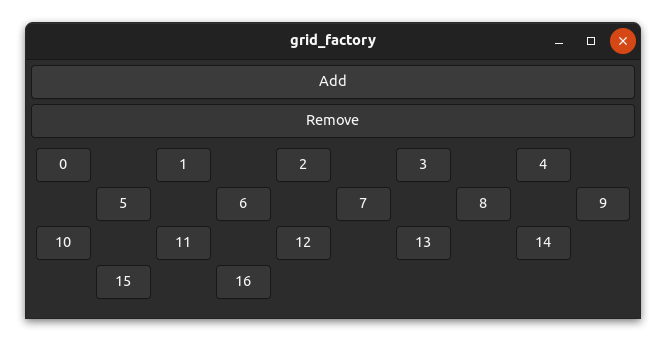# The position function

Most widgets such as `gtk::Box` don't use the position function because they are one-dimensional and place widgets relative to each other. However, a few widgets such as `gtk::Grid` use fixed positions and need the position function to work inside a factory.

The task of the position function is mainly to map the index to a certain position/area (x, y, width and height) of a factory widget within the parent widget (view).

The code we will use in this chapter is based on the grid_factory example here. Run `cargo run --example grid_factory` from the example directory if you want to see the code in action.

## How it works

Let's take a grid as an example. For a grid, there are many possibilities to place your widgets. You can, for example, place three, four or five widgets per row or you could place a certain amount of widgets per column. You can even create patterns like a chess grid if you want to.

However, we want to use a factory for generating our widgets, which means we only have the index to calculate the desired two-dimensional position. In the simplest case, we create a layout that places a certain amount of widgets per row or per column.To place three elements per row from left to right in a `gtk::Grid` we could use the following position function.

``````    fn position(&self, index: &usize) -> GridPosition {
let index = *index as i32;

let row = index / 3;
let column = index % 3;

GridPosition {
column,
row,
width: 1,
height: 1,
}
}``````

And indeed, it works as expected.## A chess grid

Let's have a look at a more complex layout. It's unlikely that this would be used in a real application, but it's still interesting to have a look at it.

To create a chess grid layout, we need to place our widgets only on fields of one color and leave the other fields empty.Actually, the code isn't too complicated.

``````    fn position(&self, index: &usize) -> GridPosition {
let index = *index as i32;

// add a new row for every 5 elements
let row = index / 5;
// use every second column and move columns in uneven rows by 1
let column = (index % 5) * 2 + row % 2;

GridPosition {
column,
row,
width: 1,
height: 1,
}
}``````

And as you can see, it works!